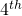# Vectors Question #1Since I’m at the topic of Vectors for most of my JC1 classes, I thought I share an interesting question regarding it. Yes, some students do tell me the questions aren’t really A’levels, but I think it does make you think and this is very important in learning.

Question: Can you place 5 points in three dimensional space such that they are pair wise equidistant?

The answer is no. Consider starting with three pairwise equidistant points with a common distant d between pairs. These are vertices of an equilateral triangle, and they will define a plane. If we add apoint that is d units from each of the other three points, then it must be placed at a distance of latex \frac{\sqrt{3}d}{2}latex 5^{th}\$ point at another location that is d from each of the other four. But we will get the same result.

If you figured this out, or understand the explanation, here is a food for thought, in 4-dimensional space, the above will possible 🙂

Not readable? Change text.